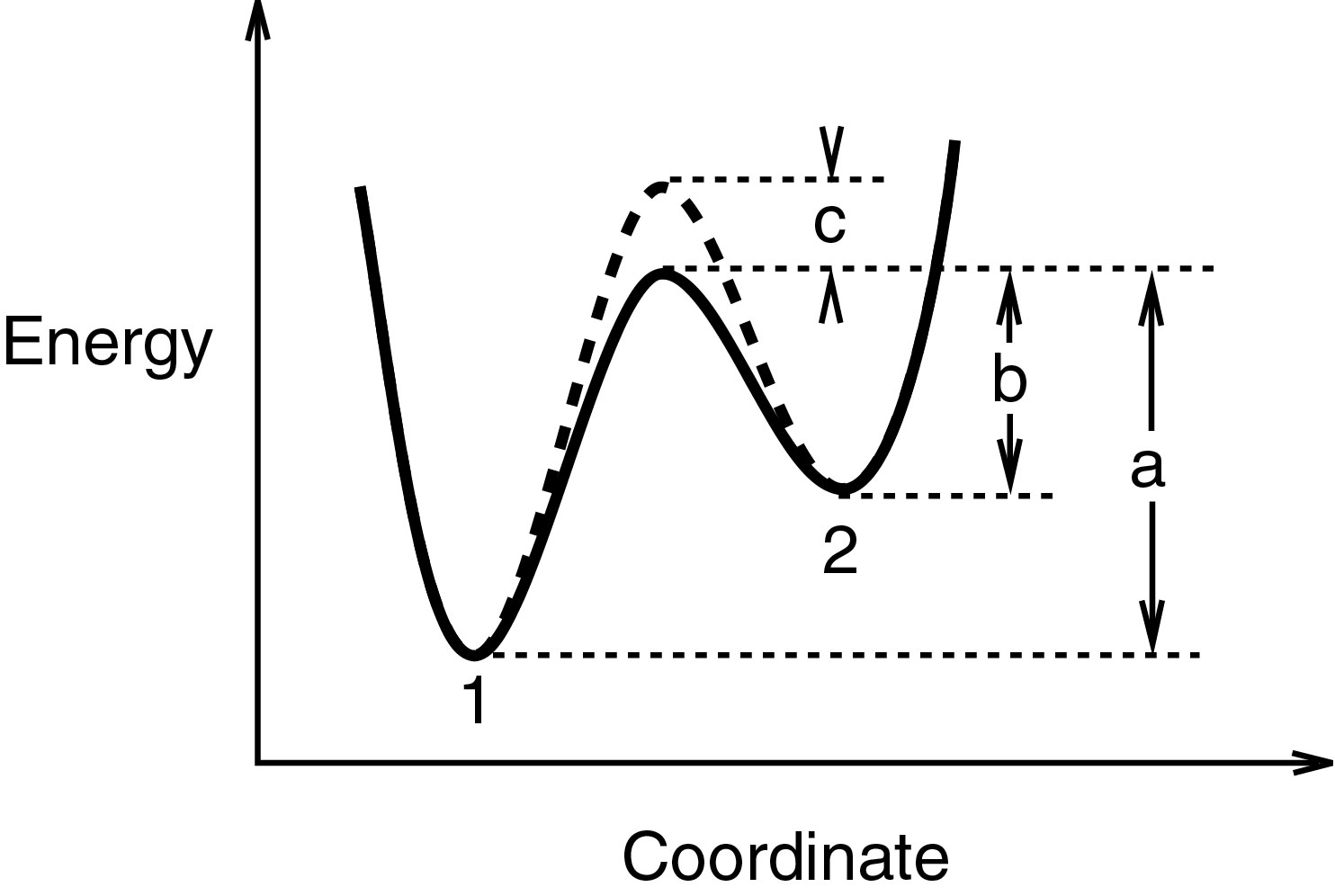A Very Simple Derivation of the Boltzmann Distribution

Consider a system at equilibrium in which particles can occupy either to two energy states, S1 or S2. The flow rate of particles from S1 to S2 will be proportional to the number of particles, n1<, in S1 and fraction of the particles in S1 with sufficient energy to get over the barrier. This fraction will be a function f(a) of the height of the energy barrier, a, that the particles must surmount in order to reach state S2,and is equal to n1f(a).Similarly, the flow from S2 to S1 will be n2f(b). At equilibrium these flows are equal, and furthermore, if the height of the barrier is increased by an amount c, the n1 and n2 will remain unchanged, and the new rates of flow will remain equal, but at different values from before. That is, that is, the two rates are n1f(a) = n2f(b) and n1f(a+c) = n2f(b+c). Dividing one by the other gives f(a)/f(a+c) = f(b)/f(b+c). The only function f satisfying this relationship is f(x) = exp(dx) where d is any constant. Thus, the fraction of particles possessing sufficient energy to cross the barrier is an exponential function of the height of the barrier and n1/n2 = exp(d(a-b)).